isMotionValid

Check if path between states is valid

Description

example

[isValid,lastValid] = isMotionValid(validator,state1,state2) checks if the path between two states is valid by interpolating between states. The function also returns the last valid state along the path.

Examples

collapse all

This example shows how to validate paths through an evironment.

Load example maps. Use the simple map to create a binary occupancy map.

map = occupancyMap(simpleMap);
show(map)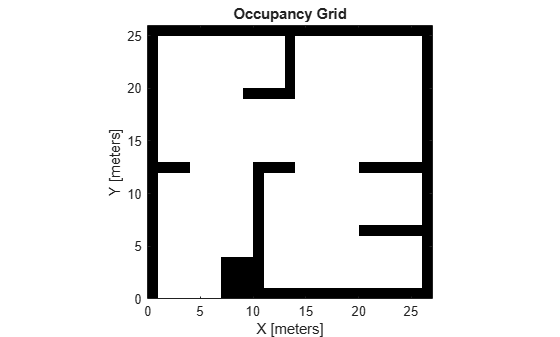Specify a coarse path through the map.

path = [2 2 pi/2; 10 15 0; 17 8 -pi/2];
hold on
plot(path(:,1),path(:,2),"--o")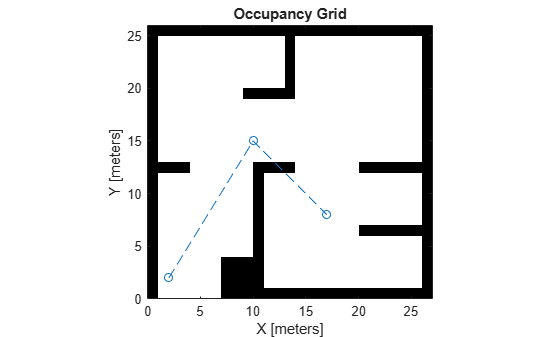Create a state validator using the stateSpaceSE2 definition. Specify the map and the distance for interpolating and validating path segments.

validator = validatorOccupancyMap(stateSpaceSE2);
validator.Map = map;
validator.ValidationDistance = 0.1;

Check the points of the path are valid states. All three points are in free space, so are considered valid.

isValid = isStateValid(validator,path)
isValid = 3x1 logical array

1
1
1

Check the motion between each sequential path states. The isMotionValid function interpolates along the path between states. If a path segment is invalid, plot the last valid point along the path.

startStates = [path(1,:);path(2,:)];
endStates = [path(2,:);path(3,:)];
for i = 1:2
[isPathValid, lastValid] = isMotionValid(validator,startStates(i,:),endStates(i,:));
if ~isPathValid
plot(lastValid(1),lastValid(2),'or')
end
end
hold off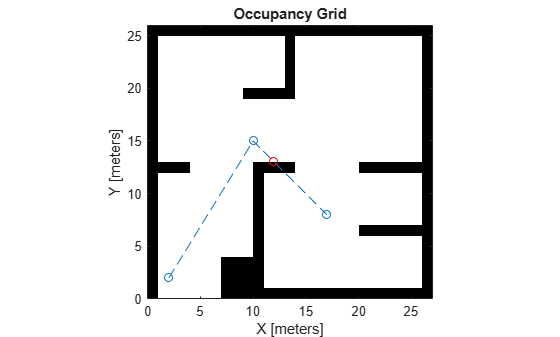This example shows how to validate paths through an evironment.

Load example maps. Use the simple map to create a vehicle cost map. Specify an inflation raidus of 1 meter.

map = vehicleCostmap(double(simpleMap));
plot(map)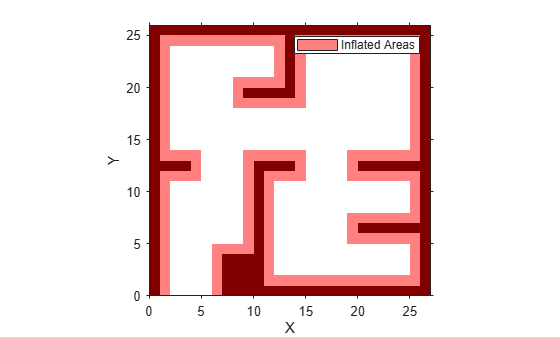Specify a coarse path through the map.

path = [3 3 pi/2; 8 15 0; 17 8 -pi/2];
hold on
plot(path(:,1),path(:,2),"--o")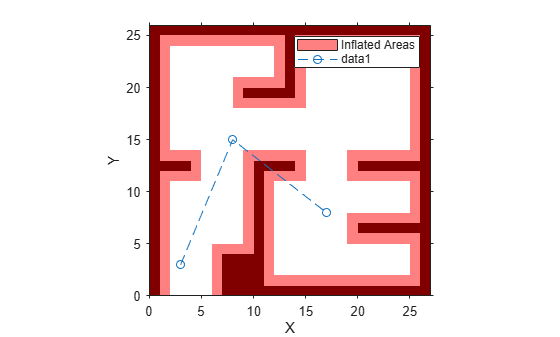Create a state validator using the stateSpaceSE2 definition. Specify the map and the distance for interpolating and validating path segments.

validator = validatorVehicleCostmap(stateSpaceSE2);
validator.Map = map;
validator.ValidationDistance = 0.1;

Check the points of the path are valid states. All three points are in free space, so are considered valid.

isValid = isStateValid(validator,path)
isValid = 3x1 logical array

1
1
1

Check the motion between each sequential path states. The isMotionValid function interpolates along the path between states. If a path segment is invalid, plot the last valid point along the path.

startStates = [path(1,:);path(2,:)];
endStates = [path(2,:);path(3,:)];
for i = 1:2
[isPathValid, lastValid] = isMotionValid(validator,startStates(i,:),endStates(i,:));
if ~isPathValid
plot(lastValid(1),lastValid(2),'or')
end
end
hold off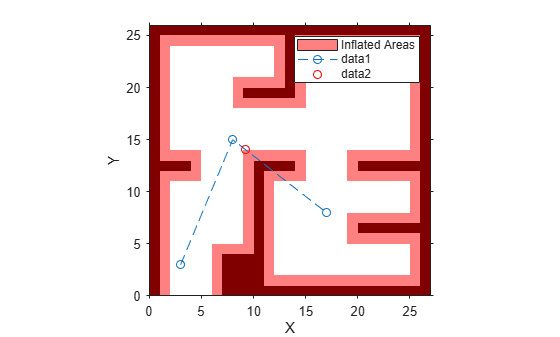Create a 3-D occupancy map and associated state validator. Plan, validate, and visualize a path through the occupancy map.

Load and Assign Map to State Validator

Load a 3-D occupancy map of a city block into the workspace. Specify a threshold for which cells to consider as obstacle-free.

omap = mapData.omap;
omap.FreeThreshold = 0.5;

Inflate the occupancy map to add a buffer zone for safe operation around the obstacles.

inflate(omap,1)

Create an SE(3) state space object with bounds for state variables.

ss = stateSpaceSE3([-20 220;
-20 220;
-10 100;
inf inf;
inf inf;
inf inf;
inf inf]);

Create a 3-D occupancy map state validator using the created state space.

sv = validatorOccupancyMap3D(ss);

Assign the occupancy map to the state validator object. Specify the sampling distance interval.

sv.Map = omap;
sv.ValidationDistance = 0.1;

Plan and Visualize Path

Create a path planner with increased maximum connection distance. Reduce the maximum number of iterations.

planner = plannerRRT(ss,sv);
planner.MaxConnectionDistance = 50;
planner.MaxIterations = 1000;

Create a user-defined evaluation function for determining whether the path reaches the goal. Specify the probability of choosing the goal state during sampling.

planner.GoalReachedFcn = @(~,x,y)(norm(x(1:3)-y(1:3))<5);
planner.GoalBias = 0.1;

Set the start and goal states.

start = [40 180 25 0.7 0.2 0 0.1];
goal = [150 33 35 0.3 0 0.1 0.6];

Plan a path using the specified start, goal, and planner.

[pthObj,solnInfo] = plan(planner,start,goal);

Check that the points of the path are valid states.

isValid = isStateValid(sv,pthObj.States)
isValid = 7x1 logical array

1
1
1
1
1
1
1

Check that the motion between each sequential path state is valid.

isPathValid = zeros(size(pthObj.States,1)-1,1,'logical');
for i = 1:size(pthObj.States,1)-1
[isPathValid(i),~] = isMotionValid(sv,pthObj.States(i,:),...
pthObj.States(i+1,:));
end
isPathValid
isPathValid = 6x1 logical array

1
1
1
1
1
1

Visualize the results.

show(omap)
hold on
scatter3(start(1,1),start(1,2),start(1,3),'g','filled') % draw start state
scatter3(goal(1,1),goal(1,2),goal(1,3),'r','filled')    % draw goal state
plot3(pthObj.States(:,1),pthObj.States(:,2),pthObj.States(:,3),...
'r-','LineWidth',2) % draw path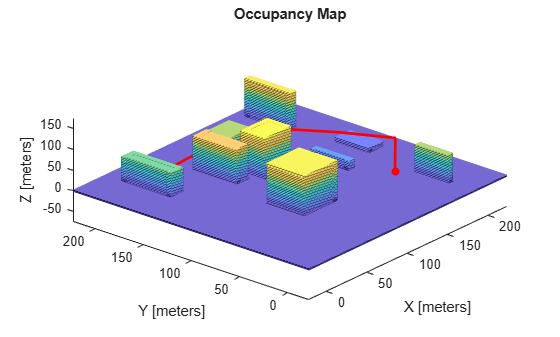Input Arguments

collapse all

State validator object, specified as an object of subclass of nav.StateValidator. These are the predefined state validator objects:

Initial state positions, specified as an n-element row vector or m-by-n matrix. n is the dimension of the state space specified in validator. m is the number of states to validate.

Data Types: single | double

Final state positions, specified as an n-element row vector or m-by-n matrix. n is the dimension of the state space specified in validator. m is the number of states to validate.

Data Types: single | double

Output Arguments

collapse all

Valid states, returned as an m-element logical column vector.

Data Types: logical

Final valid state along each path, returned as an n-element row vector or m-by-n matrix. n is the dimension of the state space specified in the state space property in validator. m is the number of paths validated. Each row contains the final valid state along the associated path.

Data Types: single | double